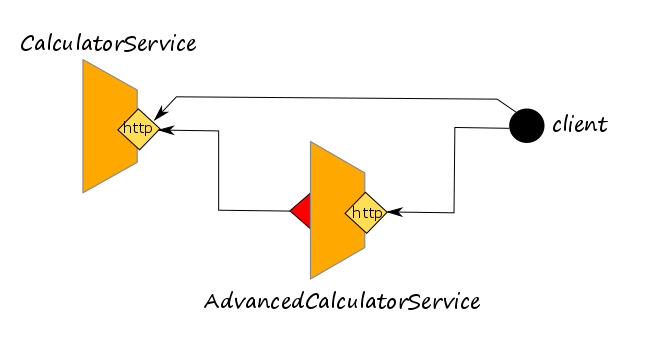# Using more than one dependency

In this tutorial we specialize the system of services presented in tutorial Using Dependencies. In particular, here we suppose to add an advertise message to each call of the `AdvancedCalculatorService`. The message is retrieved by invoking an external service not implemented in Jolie but exposed using REST.In the architecture, the `AdvancedCalculatorService` has one dependency more, from which it can get the advertise messages. In order to simulate the advertise message provider, here we exploit a funny service which returns Chuck Norris jokes.

## The new interface of the AdvancedCalculatorService

In the following, we report the new interface of the `AdvancedCalculatorService` that we modified in order to deal with the advertise messages.

``````type FactorialRequest: void {
term: int
}
type FactorialResponse: void {
factorial: long
}

type AverageRequest: void {
term*: int
}
type AverageResponse: void {
average: double
}

type PercentageRequest: void {
term: double
percentage: double
}
type PercentageResponse: double {
}

RequestResponse:
factorial( FactorialRequest )( FactorialResponse ),
average( AverageRequest )( AverageResponse ),
percentage( PercentageRequest )( PercentageResponse )
}

``````

It is worth noting that all the response messages, now contain a new field called `advertisement` that is a string. Thus we expect to receive a new advertise message for each operation call.

## The behaviour of the AdvancedCalculatorService

In the following we report the definition of the `AdvancedCalculatorService`.

``````from AdvancedCalculatorServiceInterfaceModule import AdvancedCalculatorInterface
from CalculatorInterfaceModule import CalculatorInterface

interface ChuckNorrisIface {
RequestResponse: random( undefined )( undefined )
}

execution: concurrent

outputPort Calculator {
location: "socket://localhost:8000"
protocol: http { format = "json" }
interfaces: CalculatorInterface
}

outputPort Chuck {
location: "socket://api.chucknorris.io:443/"
protocol: https {
.osc.random.method = "get";
.osc.random.alias = "jokes/random"
}
interfaces: ChuckNorrisIface
}

location: "socket://localhost:8001"
protocol: http { format = "json" }
}

main {
[ factorial( request )( response ) {
for( i = request.term, i > 0, i-- ) {
req_mul.factor[ #req_mul.factor ] = i
}
mul@Calculator( req_mul )( response.factorial )
random@Chuck()( chuck_res )
}]

[ average( request )( response ) {
{
sum@Calculator( request )( sum_res )
div@Calculator( { dividend = double( sum_res ), divisor = double( #request.term ) })( response.average )
}
|
{
random@Chuck()( chuck_res )
}
}]

[ percentage( request )( response ) {
{
div@Calculator( { dividend = request.term, divisor = 100.0 })( div_res )
mul@Calculator( { factor = div_res, factor = request.percentage })( response_mul )
response = response_mul
}
|
{
random@Chuck()( chuck_res )
}
}]
}
``````

Note that:

• there are two outputPorts definitions. The former one points to the `CalculatorService` as we described in the tutorial Getting Started, whereas the latter one points to the service `chucknorris.io` we use for simulating the advertisement service;
• the outputPort `Chuck` uses protocol `https`. The location is `socket://api.chucknorris.io:443/` where the port is the https standard one:`443`;
• the outputPort `Chuck` declares an interacted with only one operation: `random`. No types are defined.
• the HTTPS protocol has two parameters: `osc.random.method` and `osc.random.alias`. The former one specifies to use HTTP method GET when operation `random` is invoked; the latter one specifies how to build the url when operation `random` is invoked. In particular, when operation `random` is invoked, the final URL is obtained as the concatenation of the location with the specified alias(`api.chucknorris.io:443/jokes/random`). alias has been introduced in protocols `http` and `https` for mapping service operations with the actual target urls;
• in the behaviour of operation `factorial` the operation `random@Chuck` is executed after `mul@Calculator`, this means that the request message to `random@Chuck` is sent only after receiving the response from `mul@Calculator`;
• in the behaviors of operations `average` and `percentage`, `random@Chuck` is executed in parallel with those directed to service `Calculator`. Parallelism is expressed using operator `|`. A parallel composition is finished when all the parallel branches are finished. In operation `factorial` parallelism can be used too, sequential composition has been used just for illustrating a different way for composing statements;
• in the behaviour of operation `average`, the response message can be concurrently prepared in the two parallel branches because the assignments involve two different subnodes of variable `response`: `response.average` and `response.advertisement`. The parallel assignments on two separate subnodes of the same variable does not trigger any conflict;
• in the behaviour of operation `percentage`, variable `response` is not directly assigned in the response message of `mul@Calculator` ( as it happen writing `mul@Calculator( { factor = div_res, factor = request.percentage })( response )`). It is because a solicit-response always erases the variable used for storing the received reply. So, if the response to `mul@Calculator` was received after the execution of `response.advertisement = chuck_res.value` in the parallel branch, the content of node `advertisement` would be erased. Using placeholder `response_mul` and then making the assignment `response = response_mul` allows us to just valorize the root value of variable `response` preserving the contents of the subnodes.

## Running the example

In order to run the example, we need to launch both `CalculatorService` and `AdvancedCalculatorService`. Thus, we need to open two shells and run the following commands, one for each shell:

1. `jolie CalculatorService.ol`
2. `jolie AdvancedCalculatorService.ol`

In a third shell, try to run the following clients:

• `curl 'http://localhost:8001/factorial?term=5'`
• `curl 'http://localhost:8001/average?term=1&term=2&term=3'`
• `curl 'http://localhost:8001/percentage?term=50&percentage=10'`

## The complete example

The complete example can be found at this link